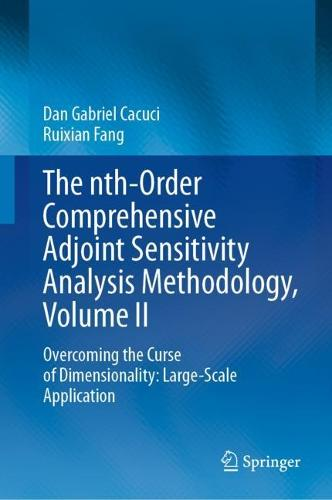•# The nth-Order Comprehensive Adjoint Sensitivity Analysis Methodology, Volume II: Overcoming the Curse of Dimensionality: Large-Scale Application (Hardback)

(author), (author)
£129.99
Hardback 463 Pages
Published: 27/04/2023
• We can order this from the publisher

UK delivery within 4-5 weeks

This text describes a comprehensive adjoint sensitivity analysis methodology (nth-CASAM), developed by the author, which enablesthe efficient and exact computation of arbitrarily high-order functional derivatives of model responses to model parameters in large-scale systems. The nth-CASAM framework is set in linearly increasing Hilbert spaces, each of state-function-dimensionality, as opposed to exponentially increasing parameter-dimensional spaces, thereby overcoming the so-called “curse of dimensionality” in sensitivity and uncertainty analysis. The nth-CASAM is applicable to any model; the larger the number of model parameters, the more efficient the nth-CASAM becomes for computing arbitrarily high-order response sensitivities. The book will be helpful to those working in the fields of sensitivity analysis, uncertainty quantification, model validation, optimization, data assimilation, model calibration, sensor fusion, reduced-order modelling, inverse problems and predictive modelling.

This Volume Two, the second of three, presents the large-scale application of the nth-CASAM to perform a representative fourth-order sensitivity analysis of the Polyethylene-Reflected Plutonium benchmark described in the Nuclear Energy Agency (NEA) International Criticality Safety Benchmark Evaluation Project (ICSBEP) Handbook. This benchmark is modeled mathematically by the Boltzmann particle transport equation, involving 21,976 imprecisely-known parameters, the numerical solution of which requires representative large-scale computations. The sensitivity analysis presented in this volume is the most comprehensive ever performed in the field of reactor physics and the results presented in this book prove, perhaps counter-intuitively, that many of the 4th-order sensitivities are much larger than the corresponding 3rd-order ones, which are, in turn, much larger than the 2nd-order ones, all of which are much larger than the 1st-order sensitivities. Currently, the nth-CASAM is the only known methodology which enables such large-scale computations of exactly obtained expressions of arbitrarily-high-order response sensitivities.

Publisher: Springer International Publishing AG
ISBN: 9783031196348
Number of pages: 463
Weight: 887 g
Dimensions: 235 x 155 mm
Edition: 1st ed. 2023